## NAME

r.accumulate - Calculates weighted flow accumulation, subwatersheds, stream networks, and longest flow paths using a flow direction map.

## KEYWORDS

raster, hydrology, accumulation, watershed, subwatershed, stream network, longest flow path

## SYNOPSIS

r.accumulate
r.accumulate --help
r.accumulate [-n0acr] direction=name format=string [weight=name] [input_accumulation=name] [input_subaccumulation=name] [accumulation=name] [subaccumulation=name] [subwatershed=name] [stream=name] [threshold=float] [coordinates=east,north[,east,north,...]] [id=integer[,integer,...]] [outlet=name] [outlet_layer=string] [outlet_id_column=name] [id_column=name] [longest_flow_path=name] [--overwrite] [--help] [--verbose] [--quiet] [--ui]

### Flags:

-n
Use negative flow accumulation for likely underestimates
-0
Use 0s instead of nulls for no flow accumulation (faster)
-a
Calculate accumulated longest flow paths
-c
Delineate streams across confluences
-r
Use recursive algorithms
--overwrite
Allow output files to overwrite existing files
--help
Print usage summary
--verbose
Verbose module output
--quiet
Quiet module output
--ui
Force launching GUI dialog

### Parameters:

direction=name [required]
Name of input direction map
format=string [required]
Format of input direction map
Options: auto, degree, 45degree
Default: auto
auto: auto-detect direction format
degree: degrees CCW from East
45degree: degrees CCW from East divided by 45 (e.g. r.watershed directions)
weight=name
Name of input flow weight map
input_accumulation=name
Name of input weighted flow accumulation map
input_subaccumulation=name
Name of input flow subaccumulation map
accumulation=name
Name for output weighted flow accumulation map
subaccumulation=name
Name for output weighted flow subaccumulation map
subwatershed=name
Name for output subwatershed map
stream=name
Name for output stream vector map
threshold=float
Minimum flow accumulation for streams
coordinates=east,north[,east,north,...]
Coordinates of longest flow path outlet point
id=integer[,integer,...]
ID for longest flow path
outlet=name
Name of input outlet vector map for longest flow path
Or data source for direct OGR access
outlet_layer=string
Layer number or name for outlet points
Vector features can have category values in different layers. This number determines which layer to use. When used with direct OGR access this is the layer name.
Default: 1
outlet_id_column=name
Name of longest flow path ID column in outlet vector map
id_column=name
Name for output longest flow path ID column
longest_flow_path=name
Name for output longest flow path vector map

## DESCRIPTION

r.accumulate calculates weighted flow accumulation, subwatersheds, stream networks, and longest flow paths using a flow direction map.

## NOTES

### Flow accumulation

Unlike r.watershed, r.accumulate does not require elevation data to calculate weighted flow accumulation. Instead, this module only uses a flow direction map to trace and accumulate the amount of flow draining through and including each cell.

With -n flag, the module will count the number of upstream cells plus one and convert it to the negative if any upstream cells are likely to receive flow from outside the computational region (flow direction edges). Negative values identify cells with likely underestimates because not all upstream cells were accounted for. Since raster map weight may contain negative flow weights, -n flag is not compatible with weight option. Running the module twice with and without -n flag and weight option may be useful in this specific case.

The module recognizes two different formats of the flow direction map: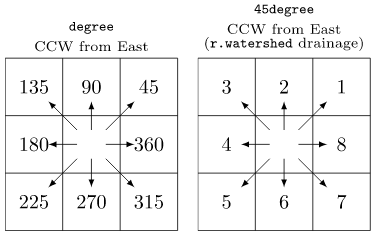Since the module does not use elevation data (i.e., slope), flow accumulation is calculated by single flow direction (SFD) routing and may not be comparable to the result from multiple flow direction (MFD) routing.

The module requires flow accumulation for any output, so it will internally accumulate flows every time it runs unless input_accumulation option is provided to save computational time by not repeating this process. In this case, it is important to use flow accumulation consistent with the flow direction map (e.g., accumulation output from this module).

### Subwatershed delineation

With subwatershed option, the module will delineate subwatersheds for outlets specified by coordinates and/or outlet options.

### Stream network delineation

With stream and threshold options, the module will delineate stream networks with the minimum flow accumulation for stream generation. A weight input map may be used with threshold. Delineated stream lines are split at confluences.

With -c flag, stream lines are delineated across confluences and may overlap with other stream lines that share the same downstream outlet.

With input_subaccumulation option, streams will be delineated as if there are no incoming flows from upstream subwatersheds defined by subaccumulation. This option is useful when you want to delineate streams completely contained inside a subwatershed without considering the main channel coming from outside the subwatershed (e.g., surface runoff within subwatersheds).

### Longest flow path calculation

With longest_flow_path option, the module will create a longest flow path vector map for outlet points specified by coordinates and/or outlet with outlet_layer option. By default, longest flow paths will be created as if there were subwatersheds at the outlets by calculating the subaccumulation map. Downstream longest flow paths will never traverse through upstream outlets. With -a flag, longest flow paths will be created in an accumulated manner resulting in an overlap between downstream and upstream longest flow paths.

You can assign unique IDs to longest flow paths using id (with coordinates) and/or outlet_id_column (with outlet). Assigning IDs also requires id_column option to specify the output column name for the IDs in the longest_flow_path vector map. The outlet_id_column specifies a column in the outlet vector map that contains unique IDs to be copied over to the id_column column in the output map. This column must be of integer type.

## EXAMPLES

These examples use the North Carolina sample dataset.

### Flow accumulation

Calculate flow accumulation using r.watershed and r.accumulate:

```# set computational region
g.region -p raster=elevation

# calculate positive flow accumulation and drainage directions using r.watershed
# for comparison, use -s (SFD)
r.watershed -sa elevation=elevation accumulation=flow_accum drainage=drain_directions

# calculate flow accumulation using r.accumulate
r.accumulate direction=drain_directions accumulation=flow_accum_new

# copy color table
r.colors map=flow_accum_new raster=flow_accum

# check difference between flow_accum and flow_accum_new
r.mapcalc expression="accum_diff=if(flow_accum-flow_accum_new, flow_accum-flow_accum_new, null())"
```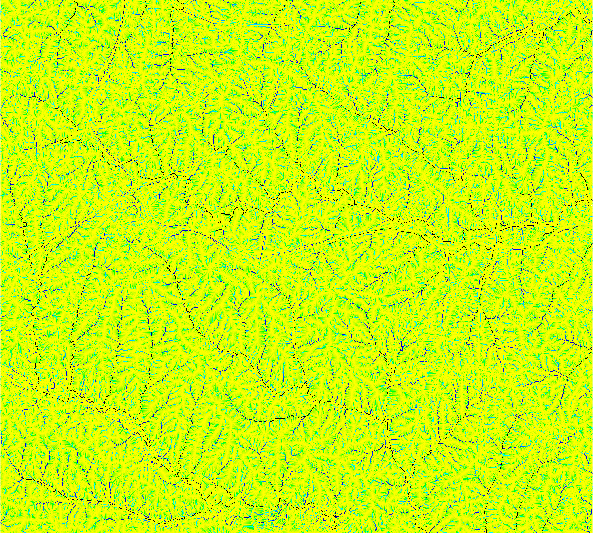For some reason, there are slight differences between the two output maps. The yellow and purple cells show the difference raster map (accum_diff). The red arrows and numbers represent drainage directions (drain_directions) and flow accumulation by r.watershed (flow_accum), respectively. Note that some cells close to headwater cells are assigned 1 even though they are located downstream of other cells.For comparison, these numbers show the new flow accumulation by r.accumulate (flow_accum_new). The same cells are properly accumulated from the headwater cells.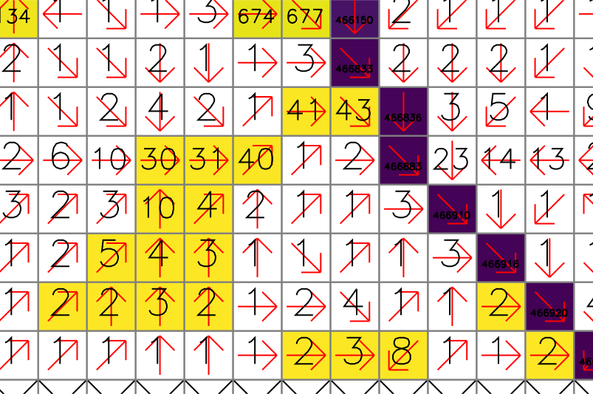### Stream network delineation

Calculate flow accumulation and delineate stream networks at once:

```# set computational region
g.region -p raster=elevation

# calculate positive flow accumulation and drainage directions using r.watershed
# for comparison, use -s (SFD)
r.watershed -sa elevation=elevation accumulation=flow_accum drainage=drain_directions

# use r.accumulate to create flow_accum_new and streams_new at once
r.accumulate direction=drain_directions accumulation=flow_accum_new threshold=50000 \
stream=streams_new

# or delineate stream networks only without creating an accumulation map
r.accumulate direction=drain_directions threshold=50000 stream=streams_new_only

# use r.stream.extract, elevation, and flow_accum to delineate stream networks
r.stream.extract elevation=elevation accumulation=flow_accum threshold=50000 \
stream_vector=streams_extract direction=drain_directions_extract
```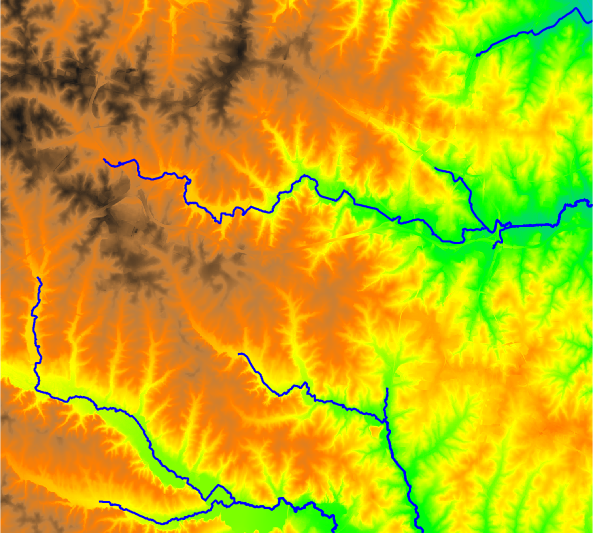In this example, r.accumulate and r.stream.extract produced slightly different stream networks where the flow turns 90 degrees or there are multiple downstream cells with the same elevation. The following figure shows an example where the streams (red from r.stream.extract) and streams_new (blue from r.accumulate) vector maps present different stream paths. The numbers show cell elevations (elevation map), and the yellow (drain_directions map) and green (drain_directions_2 map) arrows represent flow directions generated by r.watershed with -s flag and r.stream.extract, respectively. Note that the two flow direction maps are different.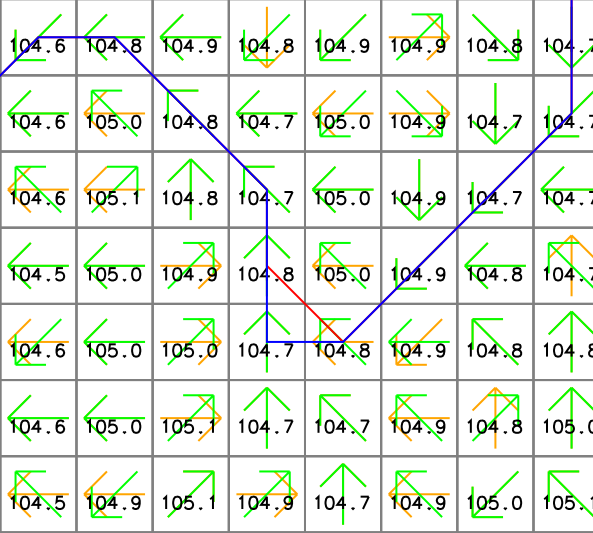### Subwatershed delineation

Delineate the watershed for one outlet:

```# set computational region
g.region -p raster=elevation

# calculate drainage directions using r.watershed
r.watershed -s elevation=elevation drainage=drain_directions

# delineate the watershed for one outlet
r.accumulate direction=drain_directions subwatershed=watershed lfp=lfp coordinates=642455,222614
```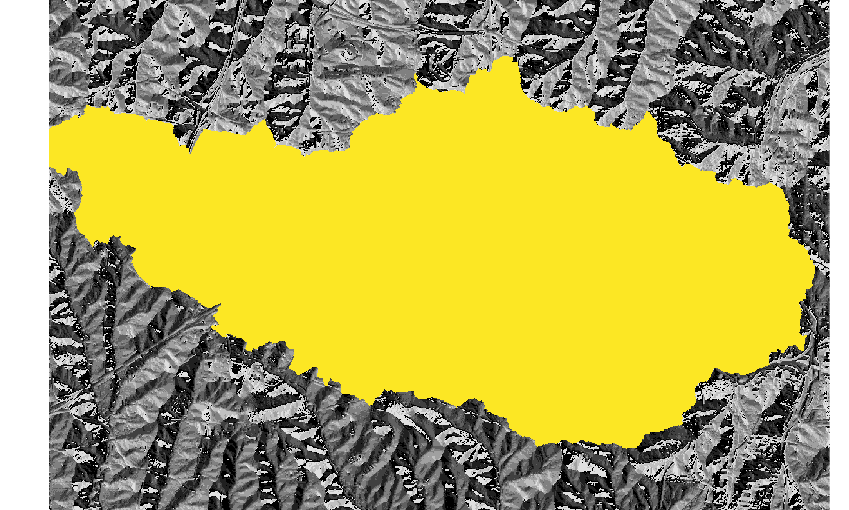Delineate multiple subwatersheds:

```# set computational region
g.region -p raster=elevation

# calculate drainage directions using r.watershed
r.watershed -s elevation=elevation drainage=drain_directions

# delineate two subwatersheds
r.accumulate direction=drain_directions subwatershed=subwatersheds \
coordinates=642455,222614,637176,223625
```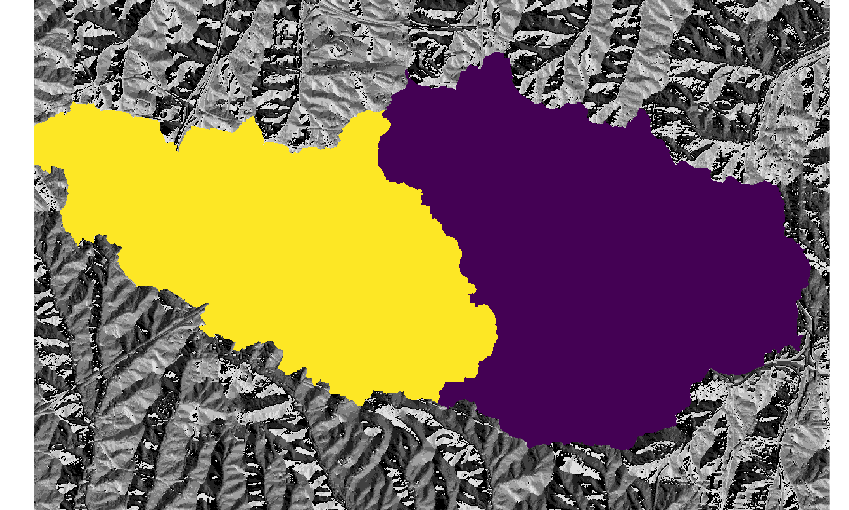### Longest flow path calculation

Calculate the longest flow path for one outlet:

```# set computational region
g.region -p raster=elevation

# calculate drainage directions using r.watershed
r.watershed -s elevation=elevation drainage=drain_directions

# calculate the longest flow path and delineate the watershed for an outlet
r.accumulate direction=drain_directions subwatershed=watershed lfp=lfp coordinates=642455,222614
```Note that there can be more than one longest flow path when multiple paths have the same flow length. In fact, the above example produces two lines with the same length.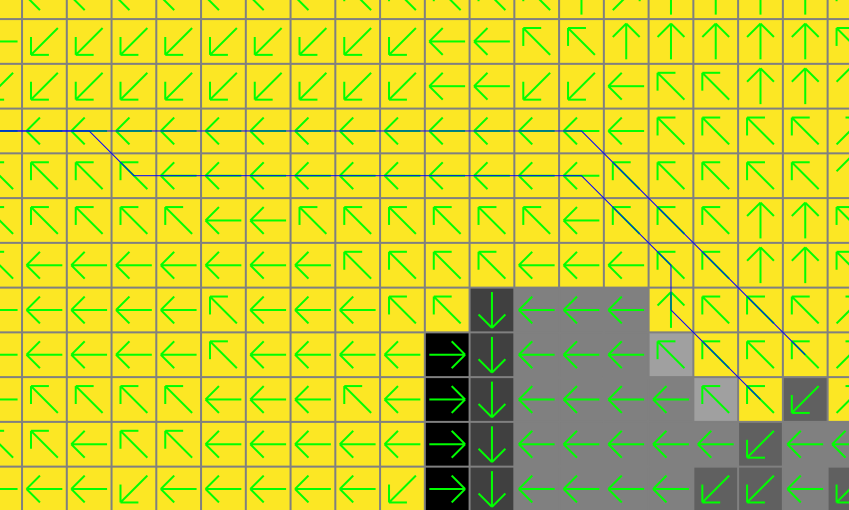There are different ways to calculate multiple longest flow paths in one run:

```# set computational region
g.region -p raster=elevation

# calculate drainage directions using r.watershed
r.watershed -s elevation=elevation drainage=drain_directions

# calculate longest flow paths at two outlets
r.accumulate direction=drain_directions lfp=lfp coordinates=642455,222614,642314,222734

# calculate longest flow paths at two outlets and assign IDs
r.accumulate direction=drain_directions lfp=lfp_w_id coordinates=642455,222614,642314,222734 \
id=1,2 id_column=lfp_id

# calculate longest flow paths at all points in the outlets map
r.accumulate direction=drain_directions lfp=lfp_at_outlets outlet=outlets

# calculate longest flow paths at all points in the outlets map and assign IDs using a column \
# in this map
r.accumulate direction=drain_directions lfp=lfp_at_outlets_w_id outlet=outlets \
id_column=lfp_id outlet_id_column=outlet_id

# calculate longest flow paths at given coordinates and all points in the outlets map and \
# assign IDs
r.accumulate direction=drain_directions lfp=lfp_multi_w_id \
coordinates=642455,222614,642314,222734 \
outlet=outlets id=1,2 id_column=lfp_id outlet_id_column=outlet_id
```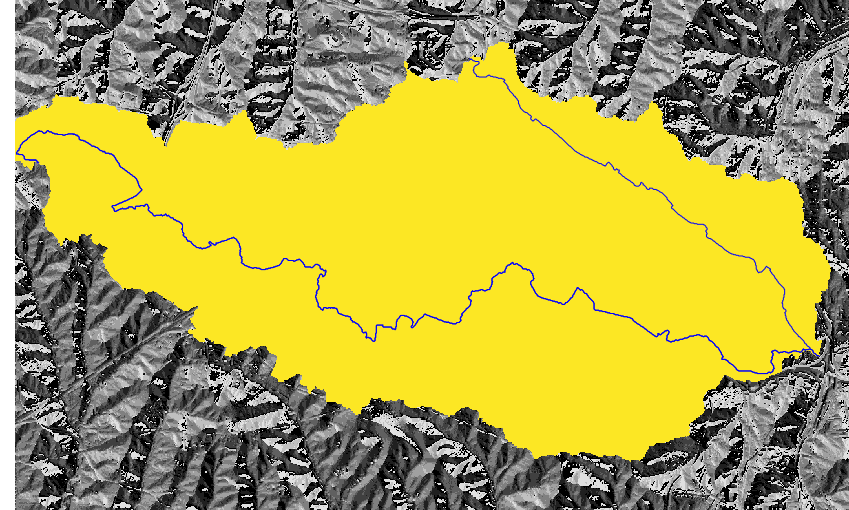### Longest flow path calculation and subwatershed delineation in one run

Calculate longest flow paths and delineate subwatersheds in one run:

```# set computational region
g.region -p raster=elevation

# calculate drainage directions using r.watershed
r.watershed -s elevation=elevation drainage=drain_directions

# get nsres
eval `r.info -g map=elevation`

# delineate streams using a threshold
r.accumulate direction=drain_directions threshold=50000 stream=streams

# populate stream lengths
v.to.db map=streams option=length columns=length

# create points along the streams starting from downstream
v.to.points -r input=streams output=stream_points dmax=\$nsres

# find outlets (downstream-most less nsres points)
cats=`db.select -c sql="select stream_points_2.cat from stream_points_2 \
inner join stream_points_1 on stream_points_1.cat = stream_points_2.lcat \
where length-along > 0.5*\$nsres and length-along < 1.5*\$nsres"`
cats=`echo \$cats | tr " " ,`
v.extract input=stream_points layer=2 cats=\$cats output=stream_outlets

# calculate longest flow paths and delineate subwatersheds for all outlets
r.accumulate direction=drain_directions lfp=lfp id_column=id \
outlet=stream_outlets outlet_layer=2 outlet_id_column=lcat \
subwatershed=subwatersheds

# convert subwatersheds to vector
r.to.vect input=subwatersheds type=area output=subwatersheds
```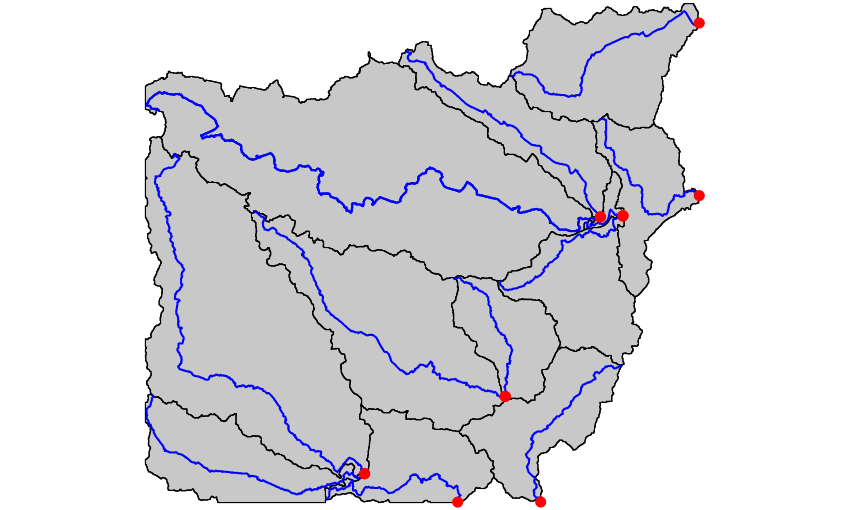r.watershed, r.stream.extract, r.stream.distance
How to delineate stream networks in GRASS GIS
How to calculate the longest flow path in GRASS GIS

## REFERENCES

Huidae Cho, September 2020. A Recursive Algorithm for Calculating the Longest Flow Path and Its Iterative Implementation. Environmental Modelling & Software 131, 104774. doi:10.1016/j.envsoft.2020.104774.

Huidae Cho

## SOURCE CODE

Available at: r.accumulate source code (history)

Latest change: Monday Jan 30 19:49:11 2023 in commit: 5fb6b1a969e72db28df78483d3360b15a17f0c68

© 2003-2023 GRASS Development Team, GRASS GIS 8.2.2dev Reference Manual